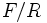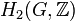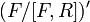# Hopf's formula for Schur multiplier

Jump to: navigation, search

## Statement

Let$G$ be a group isomorphic to the quotient group$F/R$, where$F$ is a free group and$R$ is a normal subgroup of$F$. Then, the Schur multiplier of$G$, denoted$M(G)$, which is the same as the second homology group for the trivial group action of$G$ on the integers, denoted$H_2(G,\mathbb{Z})$, is an abelian group given by the formula:$M(G) = H_2(G; \mathbb{Z}) \cong (R \cap [F,F])/[R,F]$.

In other words, it equals the quotient of the intersection of$R$ with the commutator subgroup of$F$ by the focal subgroup of$R$ in$F$. ($[R,F]$ equals the focal subgroup because$R$ is a normal subgroup of$F$).

Note that any choice of generating set for$G$ gives a choice of$F$ and$R$ for which the theorem can be applied:$F$ is the free group on those generators with the natural surjection, and$R$ is the kernel of the surjection.

## Proof

### Direct proof

Consider the short exact sequence:$1 \to R \to F \to G \to 1$

This gives a related short exact sequence:$1 \to R/[F,R] \to F/[F,R] \to G \to 1$

#### Proof of being an initial object in the category of central extensions with homoclinisms

On account of$F$ being free, this short exact sequence gives an initial object in the category of central extensions with quotient group$G$. Explicitly, for any central extension:$1 \to A \to E \to G \to 1$

there is a (unique) homomorphism$(F/[F,R])' \to E'$ that, composed with the quotient map$E' \to G'$, gives the quotient map$(F/[F,R])' \to G'$. Here is a sketch of the process:

• Consider a freely generating set for$F$. Take its image in$G$.
• For each element in the generating set for$F$, pick an element of$E$ that maps to its image in$G$.
• Consider the homomorphism$\theta:F \to E$ obtained from the set map given from the generating set of$F$ above. Note that this exists because$F$ is free.
• Verify that$[F,R]$ is in the kernel of$\theta$, so$\theta$ descends to a map$\overline{\theta}: F/[F,R] \to E$, which restricts to a homomorphism$(F/[F,R])' \to E'$.

Note that the second step of the construction introduces non-uniqueness. However, from general considerations, the map has to be unique, so this is a non-issue.

#### Consequence for the exterior square and Schur multiplier

This immediately implies that$G \wedge G \cong (F/[F,R])'$ and$M(G)$ (the Schur multiplier of$G$) is the kernel of the quotient map$(F/[F,R])' \to G'$. Let's calculate both:

•$(F/[F,R])'$ simplifies to$[F,F]/[F,R]$. Thus,$G \wedge G \cong [F,F]/[F,R]$.
• The kernel of the map from$F$ to$G$ is$R$, hence the kernel of$F/[F,R] \to G$ is$R/[F,R]$. The kernel of the map$G \wedge G \to G'$ is the intersection$([F,F]/[F,R]) \cap (R/[F,R]) = (R \cap [F,F])/[F,R]$. This, then, is the Schur multiplier.

### Proof using the Stallings exact sequence

Given: A group$G$ written as a quotient group of a free group$F$ by a normal subgroup$R$ of$F$.

To prove: Let$F' = [F,F]$ and$G' = [G,G]$. The Schur multiplier$M(G)$, defined as the kernel of the commutator map homomorphism$G \wedge G \to [G,G]$, is isomorphic to$(R \cap F')/[R,F]$.

Proof: Consider the short exact sequence:$1 \to R \to F \to G \to 1$

The corresponding Stallings exact sequence is:$H_2(F;\mathbb{Z}) \stackrel{\alpha}{\to} H_2(G;\mathbb{Z}) \stackrel{\beta}{\to} R/[F,R] \stackrel{\sigma}{\to} H_1(F;\mathbb{Z}) \stackrel{\tau}{\to} H_1(G;\mathbb{Z})$

Since$F$ is free,$H_2(F;\mathbb{Z})$ is trivial. Thus, the sequence simplifies to:$1 \stackrel{\alpha}{\to} H_2(G;\mathbb{Z}) \stackrel{\beta}{\to} R/[F,R] \stackrel{\sigma}{\to} H_1(F;\mathbb{Z}) \stackrel{\tau}{\to} H_1(G;\mathbb{Z})$

The homomorphism$\sigma: R/[F,R] \to F/[F,F] = H_1(F;\mathbb{Z})$ has kernel equal to$(R \cap [F,F])/[F,R]$. Thus, by exactness, that is also the image of$\beta$. Since$\alpha$ is trivial,$\beta$ is an isomorphism to its image. Thus, we get:$H_2(G;\mathbb{Z}) \cong (R \cap [F,F])/[F,R]$